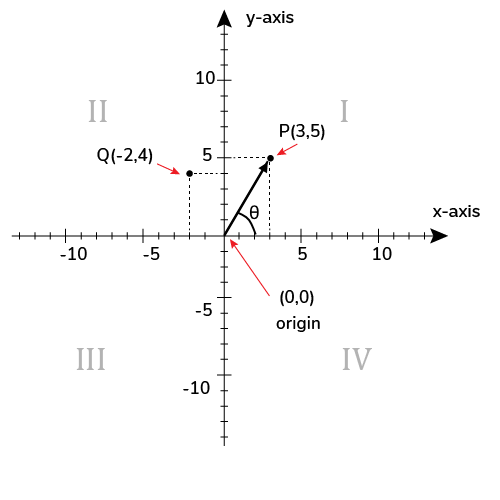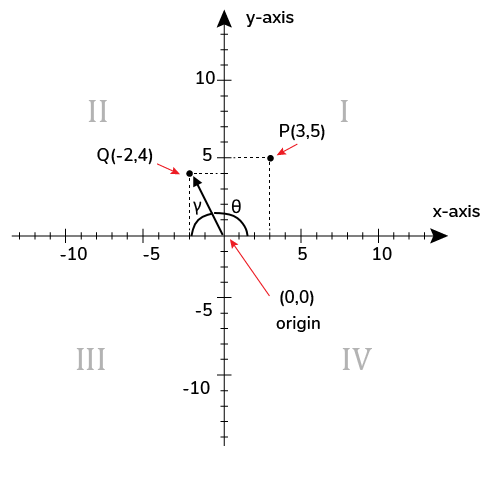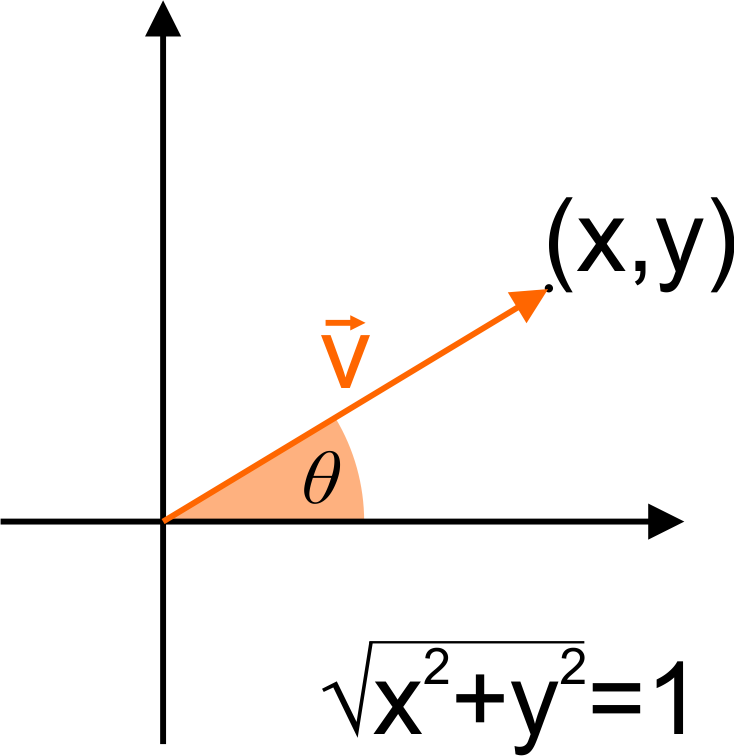# Direction of the Vector Calculator

Created by Luis Hoyos
Reviewed by Anna Szczepanek, PhD and Rijk de Wet
Last updated: Sep 22, 2022

If you want to calculate the direction of a vector, you're in the right place. This calculator finds the direction angle of a vector and calculates a unit vector in this direction.

Vectors are a powerful tool to represent many physical quantities in our physical world. They represent forces, velocities, and many other quantities derived from them.

Besides direction, finding the magnitude of the vector is also possible if you choose the advanced mode of the calculator. Therefore, with this tool, you can find the magnitude and direction angle of any vector.

## How to calculate the direction of a vector?

You can express or calculate the direction of a vector v⃗ in two ways:

1. Calculating the direction angle of the vector v⃗. The direction angle is the angle that v⃗ forms with the positive x-axis, counting in the counterclockwise direction.
2. Calculating a unit vector in the direction of the same vector. This unit vector is called the direction vector.

## How to find the direction angle of the vector?

To calculate the angle $\theta$ that a 2D vector $\vec{v} = (x, y)$ forms with the horizontal axis, use this equation:

$\theta = \arctan\left(\frac{y}{x}\right)$

The only problem with this equation is that it doesn't give us the angle about the positive x-axis, but only about the nearest horizontal axis. If your vector lies in the first quadrant of the Cartesian plane, like the vector pointing to $P(3, 5)$ in the image, that's not a problem.But what if the vector lies in any of the other quadrants? Suppose you want to find the direction angle $\theta$ of the vector $Q = (-2, 4)$ of the previous image. If we used the previous formula to find the direction angle, we wouldn't obtain the correct angle, as we'd get the angle $\gamma$ instead of the direction angle $\theta$.How can we deal with this? Well, in this case, you could have noticed that $\theta = 180^\circ - \gamma$. We can extend this reasoning to the other cases and come up with the following equations to calculate the direction of the vector in each quadrant:

• In the first quadrant, $\theta_\text{I} = \arctan(\frac{y}{x})$.
• In the second quadrant, $\theta_\text{II} = 180° - \arctan(\frac{y}{x})$.
• In the third quadrant, $\theta_\text{III} = 180° + \arctan(\frac{y}{x})$.
• In the fourth quadrant, $\theta_\text{IV} = 360° - \arctan(\frac{y}{x})$.

🙋 The term $\arctan(\frac{y}{x})$ gives an angle in radians, and you must convert it to degrees before using it in the second, third, or fourth quadrant equations. Visit our angle converter to learn how to do it.

## How do I calculate a unit vector in the direction of another vector?

To find a unit vector û in the direction of another vector v⃗ = (x, y, z), follow these steps:

1. Find the magnitude of the vector v⃗:
|v⃗| = √(x² + y² + z²)
2. Divide each coefficient of the vector v⃗ by the magnitude of v⃗:
û = v⃗/|v⃗| = (x/|v⃗|, y/|v⃗|, z/|v⃗|).
3. That's it. û is the unit vector in the direction of v⃗.

## How to find a vector of some magnitude in the direction of another?

To find a vector of a specific magnitude in the direction of another vector v⃗ = (x, y, z):

1. Find the magnitude of the vector v⃗:
|v⃗| = √(x² + y² + z²)
2. Find a unit vector û in the direction of v⃗. To do it, divide each coefficient of the vector v⃗ by the vector's magnitude:
û = v⃗/|v⃗| = (x/|v⃗|, y/|v⃗|, z/|v⃗|)
3. Multiply the magnitude of the desired vector by the unit vector û. That will result in the desired vector.

## How to find the magnitude and direction of two vectors?

To find the magnitude and direction of two vectors, you must find the resultant vector (you can use our vector addition calculator to do it) and apply to it the steps described above.

Now that you know how to find the magnitude and direction angle of a vector, let's look at some numerical examples and FAQs.

## FAQ

### How to find a vector of magnitude 3 in the direction of v = 12i - 5k?

To find a vector of magnitude 3 in the direction of v⃗ = 12i − 5k:

1. Find the magnitude of v⃗:
|v⃗| = √(12² + (-5)²) = 13
2. Find a unit vector û in the direction of v⃗. To do it, divide v⃗ by its magnitude:
û = v⃗/|v⃗| = (12/13)i − (5/13)k
3. Multiply the desired magnitude 3 by the unit vector û. We obtain the vector w⃗:
w⃗ = 3û = (36/13)i − (15/13)k
which has the desired direction and magnitude.

### How to calculate the unit vector in the direction of v = i + j + 2k

To calculate a unit vector in the direction of v⃗ = i + j + 2k:

1. Find the magnitude of v⃗:
|v⃗| = √(1² + 1² + 2²) = √6 ≈ 2.4495
2. Divide the vector v⃗ by its magnitude:
û = v⃗/|v⃗| = (1/√6)i + (1/√6)j + (2/√6)k
3. That's it. û is the unit vector in the direction of v⃗.

### Is the dot product of two vectors in the same direction positive or negative?

The dot product of two vectors in the same direction is always positive. That's because the dot product of two vectors in the same direction equals the multiplication of their magnitudes, and their magnitudes are always positive.

### How do I find the magnitude and direction of two vectors' sum?

To find the magnitude and direction of two vectors' sum:

1. Find the resultant of the two vectors.
2. Sum the square of each of the components of the resultant vector.
3. Take the square root of the previous result, and this is the magnitude of your two vectors' sum!
4. To calculate the direction of the vector v⃗ = (x, y), use the formula θ = arctan(y/x), where θ is the smallest angle the vector forms with the horizontal axis, and x and y are the components of the resultant vector.
Luis Hoyos
I want to...
normalize a vector
Find a unit vector with the same direction as your given vector.
Dimension
2DOriginal vector
x
y
Direction angle θ
deg
Unit vector
x
y
People also viewed…

### Black Hole Collision Calculator

The Black Hole Collision Calculator lets you see the effects of a black hole collision, as well as revealing some of the mysteries of black holes, come on in and enjoy!

### Log

The log calculator (logarithm) calculates the value of a logarithm with an arbitrary base.

### Quaternion

The quaternion calculator is here to deal with all your quaternion-related problems: finding the sum, difference, product, quotient, magnitude, conjugate, inverse, matrix representation, quaternion of rotation, or vector rotation.

### Significant figures

The significant figures calculator performs operations on sig figs and shows you a step-by-step solution!